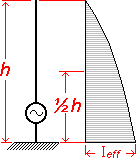Custom Search

Transmission line and antenna

Diposting oleh yc2jhz | 00.21 | | 1 komentar »

Transmission line and antenna

The characteristic impedance of a transmission line is defined as the input impedance of a line of the same configuration and dimensions but of infinite length. When a line of finite length is terminated with an impedance equal to its own characteristic impedance, the line is said to be matched.Coaxial LineThe characteristic impedance of a coaxial line (Fig. 1) is given by:

where,
4 is the characteristic impedance,
D is the inside diameter of the outer conductor,
d is the outside diameter of the inner conductor expressed in the same units as D,
kisthedielectricconstantof theinsulating material* (k equals 1 for dry air). The attenuation of coaxial fine in decibels per foot can be determined by the formula:
where,
a is the attenuation in decibels per foot of line,
f is the frequency in megahertz,
D is the inside diameter of lhe outer conductor in inches,
d is the outside diameter of the inner conductor in inches.
Parallel-Conductor Line The characteristic impedance of parallel-conductor line (twin-lead) as shown in Fig. 2 is determined by the formula:

FIG. 2

where,
Z,, is the characteristic impedance,
D is the center-to-center distance between conductors,
d is the diameter of the conductors in the same units as D,
k is the dielectric constant of the insulating material between conductors* (k equals 1 for dry air).* For a list of dielectric constants of materials, see Table 2-1. Constants and Standards ANTENNA RF Current and Voltage distribution
If a RF source is applied to a random length a - b wire then, the current distribution at the open end b will be always low and the voltage will be always high. As seen in the above graph the RF Current and Voltage distribution is divided in the wire appropriate with the physical ½ wavelength intervals

Vertical antennadl = antenna length
l = wavelengthShort antenna compared to wavelengthheff = antenna effective length
l = wavelength

ANTENNA IMPEDANCE

Z = Rs + Rls + Sx

where

Z = Antenna Impedance